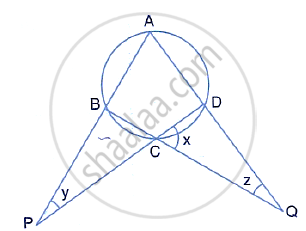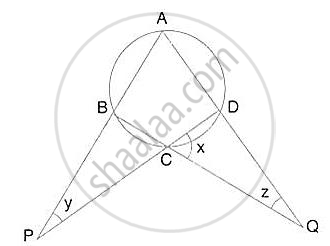Share

Calculate the Angles X, Y and Z If: X /3= Y/4 = Z/5  - ICSE Class 10 - Mathematics

ConceptArc and Chord Properties - If Two Arcs Subtend Equal Angles at the Center, They Are Equal, and Its Converse

Question

Calculate the angles x, y and z if:
x /3= y/4 = z/5SolutionLet x = 3k, y = 4k and z = 5k
∠ADB = x + z = 8k and ∠ABC = x + y = 7k

(Exterior angle of a ∆ is equal to the sum of pair of interior opposite angles)
Also, ∠ABC + ∠ADC = 180°
(pair of opposite angles in a cyclic quadrilateral are supplementary)
⇒ 8k + 7k = 180°
⇒ 15k = 180°

∴ k = (180°)/15 = 12

∴ x = 3×12= 36°

y = 4× 12 = 48°

z = 5×12 = 60°

Is there an error in this question or solution?
Solution Calculate the Angles X, Y and Z If: X /3= Y/4 = Z/5  Concept: Arc and Chord Properties - If Two Arcs Subtend Equal Angles at the Center, They Are Equal, and Its Converse.
S